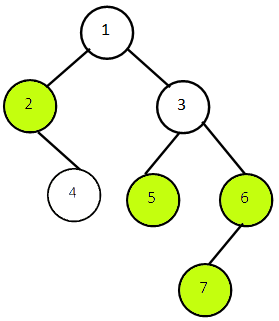# Bottom View of Binary Tree in Java

In this article, we see how to print the bottom view of Binary Tree. We discuss our approach to solve this problem and analyze the time and space complexities.

It is recommended to first learn Top View Of Binary Tree before proceeding with this problem. A lot of the implementation is based on the Top View Approach.

By Bottom View of Binary Tree, we mean the nodes visible when the tree is viewed from the bottom or from the leaves. A node is present in our bottom view it is the last node or the bottom-most node at it’s respective horizontal distance.

We calculate the horizontal distance of each node as follows:

• We fix the Horizontal Distance of Root of tree=0.
• Then, Horizontal Distance of Left Child=Horizontal Distance of it’s Parent Node – 1.
• Horizontal Distance of Right Child=Horizontal Distance of it’s Parent Node + 1.

Now let us look into an example. Consider this binary tree:The Bottom View of this tree will be : 2 5 6 7.

### Explanation

The nodes highlighted in the figure above represent the bottom view. We start from the root node 1 at level 1 the horizontal distance is fixed at 0, then we traverse each level and print the bottom-most node for that horizontal distance i.e. the node which comes last for a particular value of horizontal distance. At level 2 node 2 is the last node with horizontal distance -1 so print it. Node 5  at level 3 is the bottom-most node with horizontal distance 0. At the same level 3 node 6 is the bottom-most node with horizontal distance 3. At last level 4 node 7 has horizontal distance 2 so we print it.

Note: If one or more nodes have the same horizontal distance we consider the one’s which comes last during the level traversal.

For Example Node 1, Node 4 and Node 5 have same horizontal distance 0 but Node 5 comes later or last during level order traversal so we print node 5. Similar rule is followed for Node 3 and Node 7 having horizontal distance 2.

## Approach (Using Level Order Traversal and Hashing)

We follow these steps in order to implement this approach:

• The idea is to put the nodes with same horizontal distance(hd) together and order them on basis of their hd.
• We insert tree nodes along with their respective hd in a Queue during level order traversal by maintaining a pair class NodeObj.
• To ensure we get the bottom-most node or last node, maintain a Tree-Map of key-value pair (hd, Node-data) sorted on key hd. We keep updating and replacing the map’s key hd with the current node data until we traverse all nodes.
• For the first time, it will add a node’s hd to the map with it’s data as value, next time it will replace the value if next node’s hd is same. At the end, this make sure that the bottom-most node for that horizontal distance is present in the map.
• Then we just iterate through the Tree-Map values which will contains our Top View.

Note: We do not store node as the value in the Map only the node data is sufficient.

The Tree-Map for the above example looks like this:

 hd Node-Data -2 5 -1 10 0 25 +1 30 +2 35

So let’s look at the code of the above approach in Java.

Output:

Time Complexity: On analyzing the time complexity of our approach we see the level order traversal of all the nodes require O(n) time. Along with this, each insertion in our Map takes O (log n) time. So the Overall time Complexity will be O (n*log n).

Space Complexity:  We use a Queue that will store all the nodes present at all levels, the space required by our map would be less than our Queue so the overall space complexity will be O(n).

That’s it for the Bottom View of Binary tree you can try this approach and let us know in the comment section for any queries.